GFG App
Open AppBrowser
Continue

# Methods of Construction of Index Number

### What is Index Number?

A statistical measure that helps in finding out the percentage change in the values of different variables, such as the price of different goods, production of different goods, etc., over time is known as the Index Number. The percentage change is determined by taking a base year as a reference. This base year is the year of comparison. When an investigator studies different goods simultaneously, then the percentage change is considered the average for all the goods.

### Methods of Construction of Index Number

There are two methods through which we can construct Index Numbers: viz., Simple or Unweighted and Weighted Index Numbers.

#### 1. Unweighted or Simple Index Numbers

An Index Number in which each item must have some weight as, no weight is expressly assigned to any item is known as Unweighted Index Number. It is also known as Simple Index Number and can be constructed with the help of two techniques; viz., Simple Aggregative Method and Simple Average of Price Relatives Method.

#### i) Simple Aggregative Method:

A method in which the aggregate prices of all the selected commodities in the current year are expressed as the aggregate price of all the commodities in the base year is known as Simple Aggregative Method. It is the simplest method to construct index number. The formula for constructing index number is:#### Example:

From the following data, construct Index Numbers for 2018-2019, taking 2010-2011 as the base year by using the Simple Aggregate Method.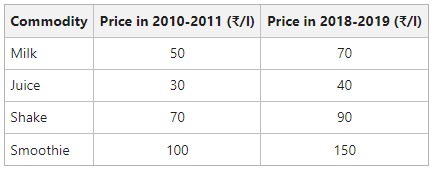#### Solution: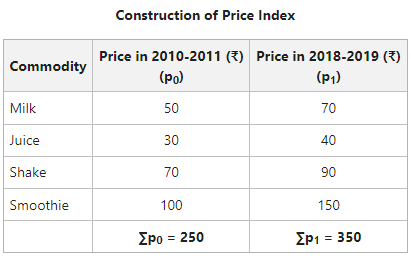Price Index for year 2018-2019 with year 2010-2011 as the base:The value of the Price Index (140) reveals that in the year 2018-2019, there is a net increase of 40% in the prices of given commodities as compared to the year 2010-2011.

#### ii) Simple Average of Price Relatives Method:

This index number is an improvement over the previous method, as it is not affected by the unit in which the prices of the commodities are quoted. The formula for constructing index number is:#### Example:

From the following data, construct Index Numbers for 2018-2019, taking 2010-2011 as the base year by using Simple Average of Price Relatives Method.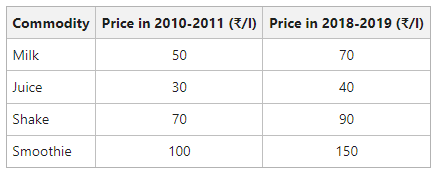#### Solution: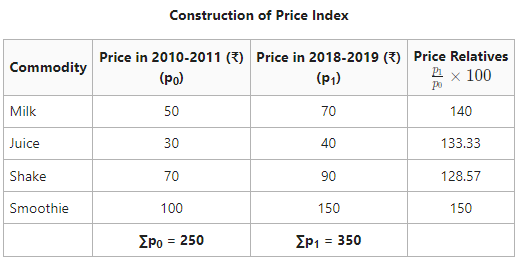The Price Index Number (137.9) shows that there is an increase of 37.9% in the prices of commodities in the year 2018-2019 as compared in the year 2010-2011.

#### 2. Weighted Index Numbers

In other index numbers, equal importance is given to each item. However, under Weighted Index Number, rational weights are given to each commodity or item explicitly. These weights indicate the relative importance of the commodities or items included in the determination of the index.

Here, rational weights mean the weights which are perfectly rational for one investigation. However, this weight might be unsuitable for other investigations. In fact, the purpose of the index number and the nature of the data concerned with it helps in deciding the rational weights.

There are two methods through which Weighted Index Numbers can be constructed; viz., Weighted Aggregative Method and Weighted Average of Price Relatives Method.

#### i) Weighted Aggregative Method:

This method involves assigning weights to different items and obtaining weighted aggregate of the prices instead of finding a simple aggregate of prices. Some important methods of constructing Weighted Aggregative Index are as follows:

• Laspeyre’s Method
• Paasche’s Method
• Fisher’s Ideal Method
• Drobish and Bowley’s Method
• Marshall Edgeworth Method
• Walsch’s Method
• Kelly’s Method

#### a) Laspeyre’s Method

The method of calculating Weighted Index Numbers under which the base year quantities are used as weights of different items is known as Laspeyre’s Method. The formula for Laspeyre’s Price Index is:#### Example:

The following are the price and quantity of four commodities A, B, C, and D, in 2015 and 2020. Construct index number of prices in the year 2020 using Laspeyre’s Method.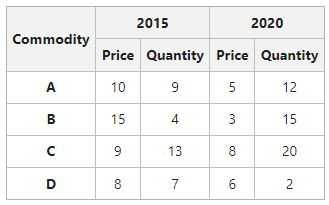#### Solution: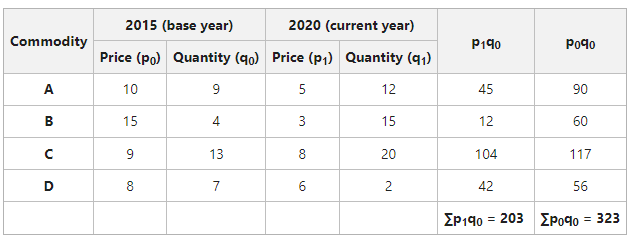= 62.84

The Index Number of Price using Laspeyre’s Method is 62.84.

#### b) Paasche’s Method

The method of calculating Weighted Index Numbers under which the current year’s quantities are used as weights of different items is known as Paasche’s Method. The formula for Paasche’s Price Index is:#### Example:

The following are the price and quantity of four commodities A, B, C, and D, in 2015 and 2020. Construct index number of prices in the year 2020 using Paasche’s Method.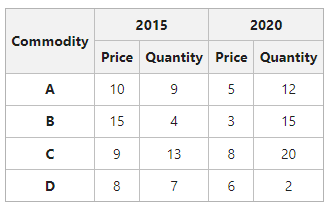#### Solution: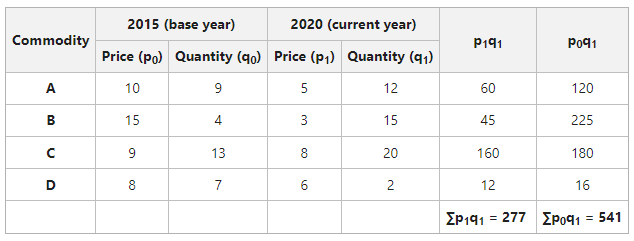= 51.2

The Index Number using Paasche’s Price Index is 51.2

#### c) Fisher’s Method

The method of calculating Weighted Index Numbers under which the combined techniques of Paasche and Laspeyre are used is known as Fisher’s Method. In other words, both the base year and current year’s quantities are used as weights. The formula for Fisher’s Price Index is:Fisher’s Method is considered the Ideal Method for Constructing Index Numbers.

#### Example:

The following are the price and quantity of four commodities A, B, C, and D, in 2015 and 2020. Construct index number of prices in the year 2020 using Fisher’s Method.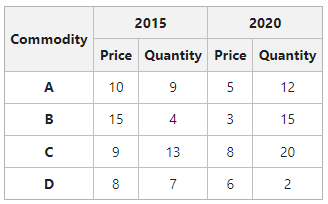#### Solution: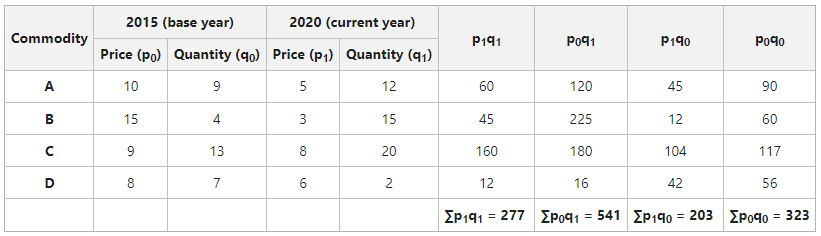= 56.72

The Index Number using Fisher’s Price Index is 56.72

#### ii) Weighted Average of Price Relatives Method:

Under this method, the base year prices of the commodities are taken as the basis to calculate the price relatives for the current year. The calculated price relatives are then multiplied by their respective weights of the items. After that, the products determined are added up and divided by the sum of weights. The formula for constructing index number is:#### Example:

Use Weighted Relatives Method and determine the index number from the following data for the year 2019 with 2008 as the base year.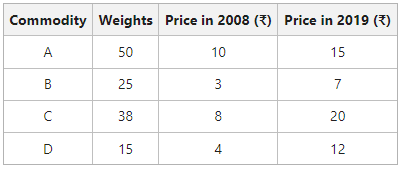#### Solution: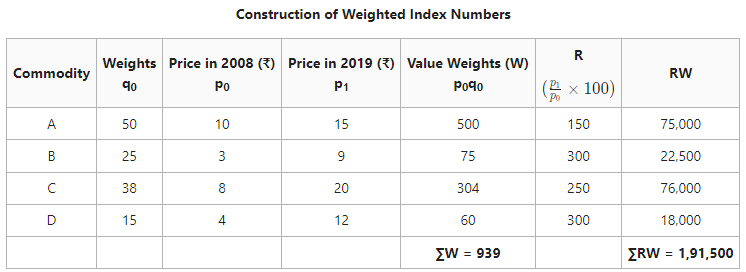Weighted Average of Price RelativesThe Index Number of 204 shows that there is an increase of 104% in the prices in the year 2019 as compared to the year 2008.

My Personal Notes arrow_drop_up